MORE SITES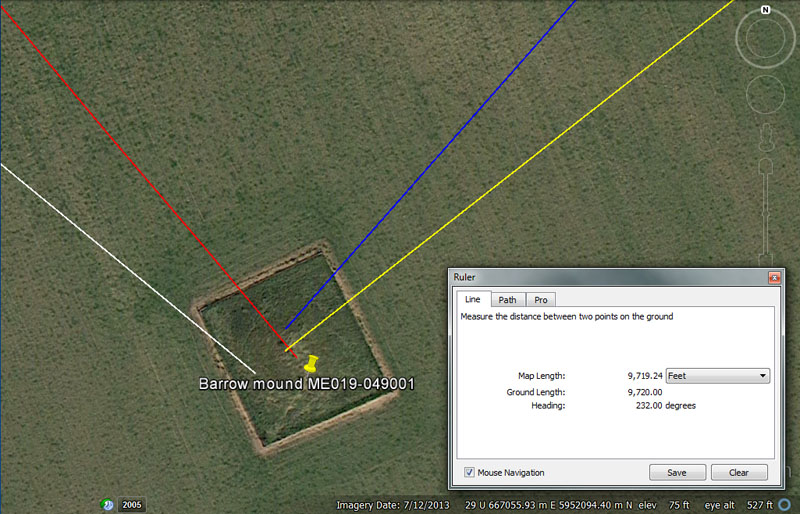Again, three of the four incoming vectors find the central region of this mound with good relative accuracy, whereas the line from Knowth chambered mound drifts slightly East of centre. This target site is very important to Newgrange chambered mound and represents the most extreme position to which the moon will move to down the horizon at lunar major standstill. At the same time, both Knowth & Dowth chambered mounds represented lunar minor standstill positions, as viewed from Newgrange chambered mound.

The white line from Knowth chambered mound runs for 6000' (also read as 6048' or 1-minute of arc) @ 129.6-degrees.

The distance aspects are self-explanatory, but the angle, 129.6-degrees was much used in ancient calculations related to the Precession of the Equinoxes, which was configured to endure for 25920-years (72-years X 360-degrees). The sum of 1296-years would be 1/20th of that long enduring cycle, where the day of the equinox changes by 1-day every 72-years and calendars have to be adjusted accordingly.

The red line from Newgrange runs at the coded distance and angle of 1900.8' @ 140 degrees.

The value 19008 is navigational coding and relates to the size of the Earth in 3 readings. Also, the ancient Roman Amphora volume for dry measures was 1900.8 cubic inches.

• The true equatorial circumference of the Earth (131383296 feet) ÷ 1900800 = 69.12. This figure (read as miles of 5280') would be 1-degree of arc for the world under the 24883.2-mile of 5280' (true size) assignment, which is only 18.8-miles in deficit of the true circumference (24902-miles).

• The "11" number family reading (league, furlong) circumference of the Earth used in navigation (130680000 feet) ÷ 1900800 = 68.75 (read as miles of 5280') would be 1-degree of arc for the world under the 24750-mile assignment. This very convenient reading was 152-miles in deficit of the true circumference.

• The "6 & 7" number families reading of the circumference of the Earth (130636800 feet) ÷ 1900800 = 68.727272 (read as miles of 5280') would be 1-degree of arc for the world under the system literally encoded int the Great Pyramid's base perimeter dimensions (where two circumnavigations is 1-minute of equatorial arc (6048'). This very convenient reading was 160.18-miles in deficit on the true equatorial circumference.
This same circumference value (130636800 feet) also equated to 24883.2-Greek miles of 5250 feet each or 12 X 12 X 12 X 12 X 1.2-miles.

It was important to have a navigational system based upon factorable number sets, which could be divided and subdivided from a whole value describing the entire equatorial circumference, down to the last inch, then subdivisions beyond that. The ancient designers of these systems would accept a small amount of error in their readings for the overall size of the Earth, but then choose a near proximity value like, 24883.2 or 24750, that was fully factorable and workable within mathematical progressions, in order to grid the world into subdivisions of the whole. By this means positional plotting became possible.
The actual discrepancy between the true size of the Earth and the factorable size nominated was negligible and had no detrimental affect upon accurate navigation across the great oceans or within the confines of the Mediterranean.

This same preoccupation with using factorable numbers only was likewise applied to reading the lunar cycles. The actual duration of the lunar nutation cycle is 6798.36-days, which is not a factorable number. However, if the cycle is mathematically described as 6804-days (5.64-days in excess), then this long enduring cycle can be broken down into lunar years, lunar months, weeks, days, etc., to the last second in a 24-hour day.
By this method the moon's position in relation to the Earth can be tracked with excellent relative accuracy on a day-to-day basis, and tiny adjustments can be made infrequently to achieve full precision, as required.

The angle of 140-degrees provides a multi-use number much used in all manner of navigational and calendar computations.

The blue line from Dowth chambered mound runs for 6336' (1.2-miles) @ the coded angle of 41.472-degrees return (41 & 59/125ths). Obviously, this is only the target value or finalised coded number, but orienteers could find the target by rounding the angle to 41.5-degrees. However, it was essential to know the full significance of 41472 and how it is generated within a mathematical progression.

Of course, the distance value of 6300' (1.2 Greek miles) would have been the main distance code for orienteers navigating overland from Dowth mound.

The 6336' distance is navigational coding related to readings of the equatorial circumference in Egyptian Royal Cubits:

• The true equatorial circumference of the Earth (131383296 feet) ÷ 6336 = 20736. This figure (read as 20.736 inches) was length of the Egyptian Royal cubit, which mnemonically coded the 24883.2-mile (of 5280' each) assignment. This was only 18.8-miles short of the true equatorial circumference. It's very evident in assessing ancient French measurements that they held to a mathematical concept of the world being 24883.2-miles of 5280', based upon their "Lieue de 25 au degré" tradition. This states that 1-degree of arc was 2282 Toise and the drifted Toise, was by 1669, about 6.394'.
• The "11" series (league, furlong) circumference of the Earth (130680000 feet) ÷ 6336 = 20625. This figure (read as 20.625 inches) was the length of the Egyptian Royal Cubit, which mnemonically coded the 24750-mile (of 5280' each) assignment.

• The "6 & 7" series (yard or reed) circumference of the Earth (130636800 feet) ÷ 6336 = 20618.18182. This figure (read as 20.61818182 inches) was the length of the Egyptian Royal Cubit, which mnemonically coded the 24741.81818-mile (of 5280' each) assignment. Note that this value, when reduced to feet (130636800') = 24883.2 Greek miles.

Of interest is the fact that the Roman Modius volume for dry measure was 633.6 cubic inches.

The 41.472-degree angle is navigational coding also and the sum of 41.472-miles would be 1/600th of the 24883.2-mile equatorial circumference.

The yellow line from Dowth Henge's centre runs for 6720' (1 ancient Irish mile) @ 52-degrees return.

A length of 6048' (1-minute of equatorial arc) is .9 of 6720'. The Alexandrian Mina weight was 6720-grains.

The return angle of 52-degrees provides a value much used in calendar computations, thus 52-weeks in a solar year.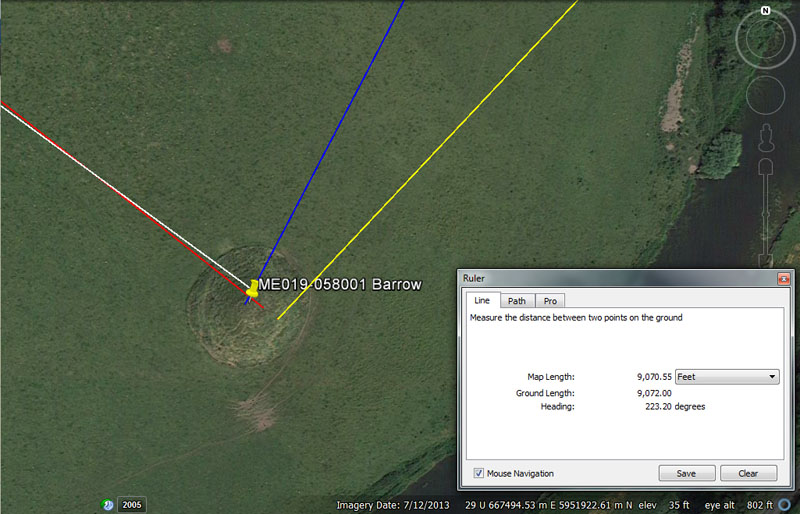Adjacent to the Boyne River is this large mound with internal chambers, which could also have offered accommodation for orienteers out-&-about doing their assigned explorations. This very important mound marked the rise position of the sun at the Winter solstice from Newgrange passage.

(1). The distance and angle from Knowth mound (white line) was 7500' @ 126-degrees.

A distance of 52500' (10 Greek miles) would be 7500' X 7.

The angle value accentuates one of the most important numbers of antiquity and the Greek system of metrology was based upon a foot of 12.6". There would be 126" in a Hebrew reed (10.5'). The duration of the lunar nutation cycle (6804-days) = 54 X 126-days. The 2551.5-day lunar period tracked within the Sabbatical Calendar (7.2 lunar years) is 126 X 20.25-days (486-hours), etc

In 606 B.C. Nebuchadrezzar II restored the ancient Sumerian weights system used 1500-years prior to his reign and amongst these was a shekel weight of 126 grains. This was used in the gold standard for measuring out quantities of the precious metal and the weight was later adopted by Charlemagne in 789 AD.

(2). The distance and angle from Newgrange (red line) was 3360' @ 128-degrees.

This distance is, of course, half an ancient Irish mile of 6720'.

The angle that orienteers from Newgrange followed to encounter this mound target (128-degrees) is in homage to 8, 16, 32, 64, 128. etc., and in halving, quartering, etc., the 360-degree compass, which has been the standard modus operandi for compass readings since time immemorial.

(3). The distance and angle from Dowth mound (blue line) was 6048' @ 207.36-degrees.

The distance is, of course, 1-minute of equatorial arc for the world under the 5250-mile reading and would also have been read as 6050' to fit the 5280' mile.

The degree angle also relates to the size of the Earth and 207.36-miles would be 1/120th of the 24883.2-mile equatorial circumference or 3 arc-degrees.

(4). The distance and angle from Dowth Henge (yellow line) was 9072' @ 43.2-degrees.

The distance is 1.5 minutes of equatorial arc and would also have been read as 9075'. The Great Pyramid @ 756' of length is 9072" long. Speaking further of Charlemagne's weight standard, his reintroduced Talent had originally been 907200-grains in 2100 BC.

The angle of 43.2-degrees return is in homage to the very useful value of 432, used in many ancient calculations. The Great Pyramid @ 756' is 432 Celtic or Hebrew Royal Cubits (of 21" each) long. The Precession of the Equinoxes (25920-years) is 432 X 60-years in duration.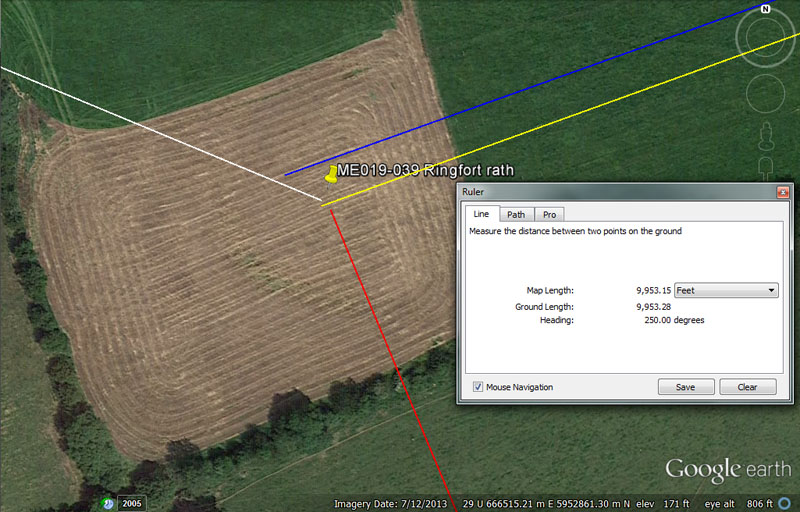The faint outline of this ringfort rath can still be seen in the landscape.

(1). It sits 3200' from Knowth chambered mound (white line) @ 112.5-degrees.

Both the distance and the angle offer a tutorial in dividing up the 360-degree compass into 32 or 320 divisions, based upon 11.25-degrees or 1.125-degrees.

The angle of 112.5 is one of the primary angles achieved when dividing the 360-degree compass into 32-divisions.

(2). It sits 1215' from Newgrange chambered mound (red line) @ 337.5-degrees.

The 1215' value is in homage to the moon and there would be 56 intervals of 121.5-days in the 6804-day lunar nutation cycle, which would also explain why there were 56-posts on the Aubrey Circle at Stonehenge.

Again, the angle of 337.5-degrees is one of the primary angles generated when dividing up the 360-degree compass into 32-divisions.

(3). It sits 6300' from Dowth chambered mound (blue line) @ 250-degrees.

This distance is, what much later in history became, 10 Greek Stadia. This same distance is encoded into the North American landscape between the geometric earth embankment structures of the Octagon of Newark, Ohio and the Great Circle embankment nearby (centre-to-centre). The inner rim circuit at Stonehenge mnemonically represented both 314.16' (PI) of circumference or 315' (half a stadia ... based upon a rounded form of PI).

The value 250 is, of course, accentuating increments in 1000s or decimal divisions. The Romans, perhaps, using an alternative foot of 11 & 2/3rds inches, with 5000 such feet making a mile, configured the equatorial circumference to be 75 such miles for 1-degree of arc. This equates to 131250000 standard feet of 12" or 25000 Greek miles or 5000 Egyptian Aturs.
Whether or not such an added system was ever used in navigation remains to be seen. It is hypothetically possible and mathematically plausible, but unproven and the known Roman mile was 4860' or 5000 Roman feet of 11.664".

(4). It sits a coded distance of 9953.28' from Dowth Henge (yellow line) @ 250-degrees.

This is the same coded distance that exists between the centre of Dowth Henge and the centre of Newgrange chambered mound. The sum of 9953.28-miles X 2.5 = 24883.2-miles (the equatorial circumference of the Earth under, what much later became, the Greek navigational method using a mile of 5250' ... or 1/5th of an Egyptian Atur).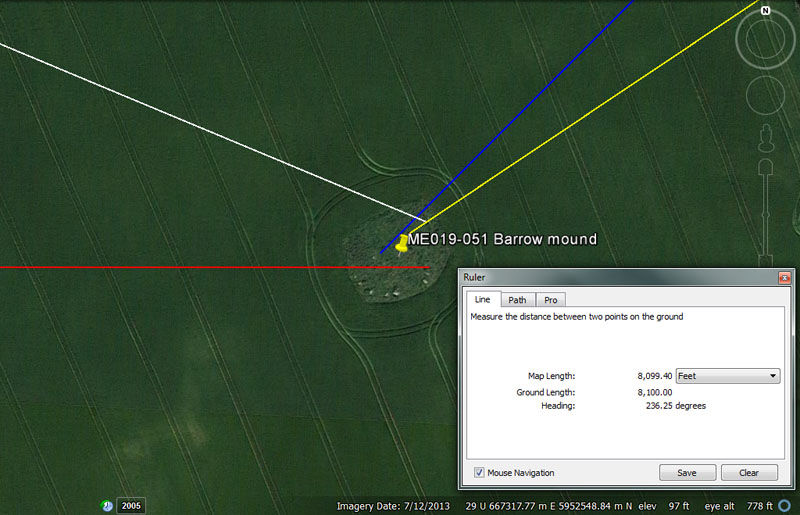This mound also contained an inner chamber where orienteers could shelter and get a good night's sleep out of the wind and cold.

(1). From Knowth chambered mound (white line) the distance is 6048' @ 112.5-degrees.

This distance is 1-minute of equatorial arc for the world and would be equivalent to traveling twice around the base perimeter of the Great Pyramid (@ 756' per side). Of course it could also have been read as 6050', or 1-minute of arc under the navigational method that used the 5280' & 5940' miles.

The degree angle is, yet again, one of the primary segmentations achieved when dividing the 360-degree compass into 32 divisions.

(2). From Newgrange chambered mound (red line) the distance is 2160' @ 90-degrees.

In the 25920-year duration of the Precession of the Equinoxes, the sun spends 2160-years in each of the 12-houses of the zodiac. Likewise, the original English bushel was 2160 cubic inches or 1/10th of the Babylonian Homer volume (21600 cubic inches) The English bushel would also be 100 Hebrew Desert Standard Logs of volume @ 21.6 cubic inches each. Likewise, in the 12 X 12 X 12 X 12 X 1.2-mile (of 5250' each) circumference of the Earth (130636800') the sum of 6048' (1-minute of arc) would be 1/21600th part. The moon is 2160-miles in diameter.

(3). From Dowth Mound (blue line) the distance would be read as 4666.6666' (4666 & 2/3rds) or 4665.6' @ 225-degrees.

The Roman Christian church fathers, when they got a foothold in certain countries, chastised the late era Druidic (so-called pagans, paysans-pagani or peasants) savants for their use of the 666 combination of numbers and demonised the practice, with 666 being called the devil's number and indicative of dabbling in evil.
The ancient mathematicians, as well as much later counterparts, used a variety of such numerical sequences, such as 10.66666 (once the basis for an early French standard foot @ 1.06666 feet), 11.66666" (which, undoubtedly, became a slight variation on the Roman foot), 14.66666, 16.6666, 18.6666, etc., as they were very useful in all manner of calculations.

This length extending from Dowth chambered mound, equates to 4800 so-called Roman feet of 11.664" or 4665.6' and would equate to 1/28000th of the equatorial circumference of the Earth under the 5250-miles navigational system.

Again the degree angle is a primary division of a 360-degree circle divided into 32 parts.

(4). This structure sits 8100' from the centre of Dowth Henge (yellow line) on an outrunning angle of 236.25-degrees and return angle of 56.25-degrees.

The distance aspect is in homage to rounded PHI and 2 X 8100' = 16200'. The pure PHI ratio is 1: 1.6180339 and the distance would also have been used in tutorials related to .80901695 or the half PHI value, used in the design geometry of four of Stonehenge's concentric circles.

A thorough understanding of the PHI ratio and how to manipulate it in calculations was central to successful ancient society, especially in relation to correct volume measures of dry goods sold in marketplaces. The PHI ratio, half-PHI and the PHI reciprocal constituted, arguably, the most important knowledge of ancient scientists-mathematicians and the concept of civilisation was incomplete without this knowledge.

It was essential to know how to square the circle, using the PHI ratio and, especially, the PHI reciprocal, in order to fabricate round measuring tubs of perfect cubic inch capacity for dispensing quantities of grain, etc., in ancient marketplaces.

The 8100 value is multifunctional and much used in a mathematical progression generating values for tracking the period of the moon. For example, the 6804-day lunar nutation cycle is 81 intervals of 84-days, or the 2551.25-day period of the moon (7.2 lunar years), daily monitored within the lunisolar calendar, is 81 intervals of 31.5-days (756-hours).

The angle of 236.25-degrees is part of a lunar mathematical progression and the lunar year (354.375-days) is 236.25-days X 1.5. The value is equally important for time interval divisions within the 6804-day lunar nutation cycle.

The 56.25-degree return angle is, yet again, one of the angles achieved when dividing the 360-degree compass into 1/32nd divisions, as mariners and others have done since time immemorial.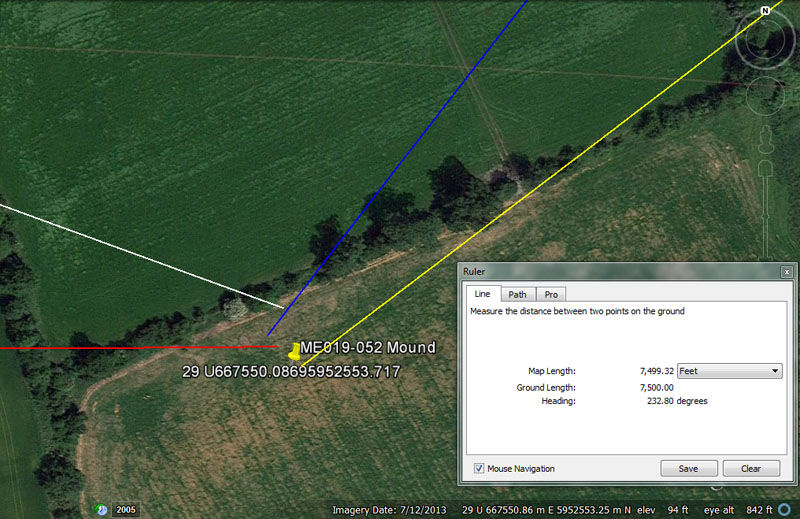The archaeological record places a mound at these coordinates, although the structure cannot be positively identified within the Google Earth image.

(1). From Knowth (white line) the coded distance and angle appear to be 6720' (1-Irish mile) @ 110-degrees.

The distance is self explanatory and an increment of 6720' represents a perfect, whole number division of the 24883.2-mile (of 5250') equatorial circumference or 1/19440th of that circuit. Under this navigational system, there are 66 & 2/3rds seconds of arc (@ 100.8' per arc second) in 6720'.

The angle accentuates the number 11, which was the base value for the ancient English league @16500' (1500 X 11), the 5940'-mile (540 X 11), the 5280'-mile (480 X 11), the furlong or furrowlong @ 660' (60 X 11), the rod/ perch @ 16.5' (1.5 X 11) or the original fathom @ 5.5' (.5 X 11). Under this very much-used navigational system the equatorial circumference was deemed to be 24750-miles of 5280' each or 130680000'.

(2). From Newgrange Mound (red line) the distance is 2880' @ 89.6-degrees.

The 288 value was much used in ancient calculations and we can deduce, from the writings of Greek historian Herodotus, that the Egyptian Pyramid acre was 28000 square feet (of 12" each). Likewise, this distance of 2880' would be 1/5th of what researcher John Michell dubbed the "Geomancer's-mile" of 14400', an increment found to be positively identifiable between structural positions on many ancient sites.

In the 24883.2-mile equatorial circumference of the Earth, the sum of 28.8-miles (151200' or 25-minutes of arc) would be 1/864th segment. Remember, the distance from the centre of Dowth Henge due North (0-360-degrees) to the summit cairn of Slieve Gullion Mountain is 151200'.

The angle of 89.6-degrees is also navigational coding under the 24883.2-mile reading of the Earth's equatorial circumference and 89600' would be 1/1458th segment. Also, the sum of 89.6' would equate to 1/75th of the ancient Irish mile of 6720'.

(3). From Dowth Mound (blue line) the intended, coded-distance reading is 4147.2' @ 217.8-degrees.

The value 4147.2 was, once again, related to a perfect division of the equatorial circumference and 4147.2-miles would be 1/6th of the 24883.2-mile distance.

In the same vein, the degree angle encodes a segment of the equatorial circumference under the 24750-mile (of 5280') equatorial-circumference, navigational system (130680000'). Under this system the sum of 2178' would be 1/60000th of the circuit around the Earth and the sum of 217800' would equate to 36 minutes of arc.

(4). From Dowth Henge (yellow line) the distance is 7500' @ 52.8-degrees return.

A mile of 5250' equates to .7 of 7500'. Likewise, if the distance was read as 7560', that would constitute 1.25-minutes of equatorial circumference arc under the navigational system that used the 5250'-mile.

For readings related to the navigational system that used miles of 5280' or 5940', 1.25-minutes of arc would be 7562.5'.

The coded angle of 52.8-degrees is in homage to the 5280' mile.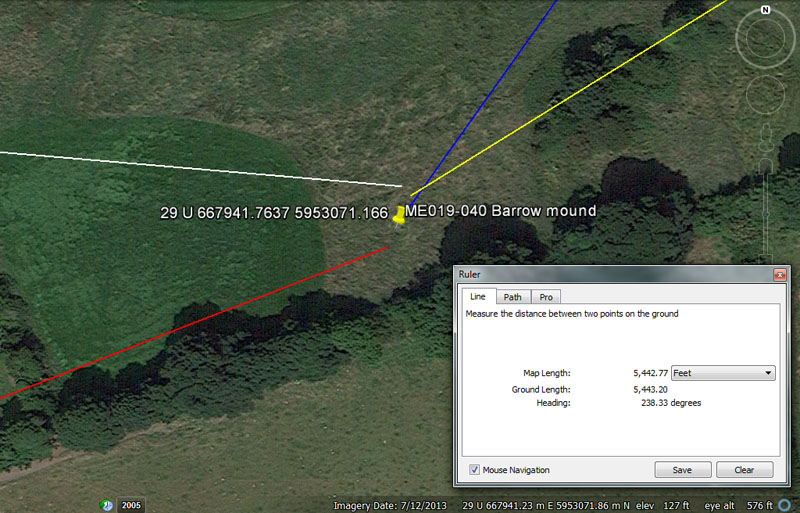(1). From Knowth chambered mound (white line) the distance is 7700' @ 95.04-degrees.

The sum of 7700' is 1400 fathoms of 5.5' each. If this distance was symbolically read as 7776' for tutorial purposes, it would relate to the 24883.2-mile equatorial circumference, of which 777.6-miles would be 1/32nd part.

The degree angle is also navigational coding under the 24750-mile (of 5280') equatorial circumference (130680000') and, accordingly, the sum of 95040' (18-miles) would be 1/ 1375th part.

(2). From Newgrange chambered mound (red line) the distance is 4536' @ 68.75-degrees.

The distance is, again, navigational coding and the sum of 4536' equates to .75 of 1-minute of equatorial arc under the 24883.2-mile (of 5250') system. This measurement equates to 240 Egyptian Royal cubits of 20.618" (an example of which is in the Turin Museum). The way to find the exact length of this cubit is to divide the base length of the Great Pyramid by 440. Therefore, 756' (9072") ÷ 440 = 20.61818182" (20 & 34/55th).

Under this system the sum of 45360' would be 1/2880th of the equatorial circumference or 7.5 arc minutes.

It is evident that the Great Pyramid never had a pointed top but was designed to have a flat-floor altar or observation platform at a height of 453.6'.

The degree angle is also navigational coding and 68.75-miles (of 5280') would equate to 1-degree of arc under the 24750-mile equatorial circumference navigational method.

(3). From Dowth chambered mound (blue line) the distance is 2016' @ 35.4375-degrees return.

This distance is 1/3rd of 1 arc minute under the 24883.2-mile (of 5250') navigational method, whereas the sum of 2016.6666' would be 1/3rd of 1 arc minute under the 24750-mile (of 5280') method.

The coded degree angle is lunar and the sum of 35.4375-days (35 & 7/16ths) is 1/10th of the lunar year (354.375-days).

(4). (2) From Dowth Henge (yellow line) the distance is 5443.2' @ 58.32-degrees.

The 5443.2 coding is navigational and relates to the size of the Earth under the 24883.2-mile (of 5250') navigational system (130636800') for which the sum of 54432' would be 1/2400th part or 9 arc minutes of equatorial circumference.

The angle of 58.32-degrees is also navigational and the sum of 58.32" became the Roman pace increment (5 Roman feet of 11.664") about 2700 years after these distances and angles were encoded into the Boyne River valley landscape.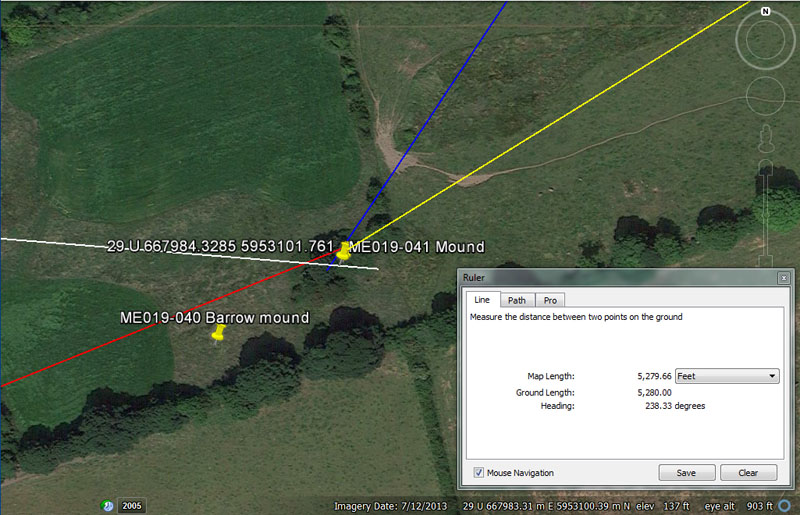From Knowth (white line) the distance is 7875' @ 94.5-degrees.

This distance is 1.5 miles (of 5250').

The angle carries both navigational and lunar coding simultaneously and the sum of 94.5' would be 1/64th of 1 arc minute (6048'). Likewise, the sum of 94.5-days would equate to 1/72nd of the 6804-day lunar nutation cycle, as well as 1/27th of the 2551.5-days (7.2 lunar years) tracked within the lunisolar Sabbatical calendar system alongside 7 solar years (2556.75-days).

From Newgrange chambered mound (red line) the distance is 4725' @ 68.04-degrees.

The distance is lunar coding and there would be 7.5 intervals of 47.25-days (1134-hours) in a lunar year (354.375-days ... 8505-hours). There would also be 144 intervals of 47.25-days in the 6804-day lunar nutation cycle or 54 such intervals in the 2551.5-day count of the lunar period within the lunisolar calendar.

The degree angle, of course, is in homage to the 6804-day lunar nutation cycle.

From Dowth chambered Mound (blue line) the distance is 1890' @ 33.75-degrees.

The sum of 189' would equate to 1/4th the length of the Great Pyramid or 1/32nd of 1 arc minute (6048') and 1890' X 192 would equal 1-degree of arc (69.12-miles of 5250' each) under the 24883.2-mile navigational method.

The degree angle coding is both navigational and lunar coding simultaneously and, when dividing the 360-degree compass into 32-parts, 3/32nds = 33.75-degrees and 15/16ths = 337.5-degrees. There would be 10.5 intervals of 33.75-days (810-hours) in a lunar year of 354.375-days).

From Dowth Henge (yellow line) the distance is 5280' @ 58.32-degrees (alternative reading 58.3333)

The distance is, of course, what later became known as the English mile.

The degree angle relates to what became known as the Greek mile (58.33333 X 90 = 5250') and the Roman mile ... 58.32 X 83.33333 = 4860').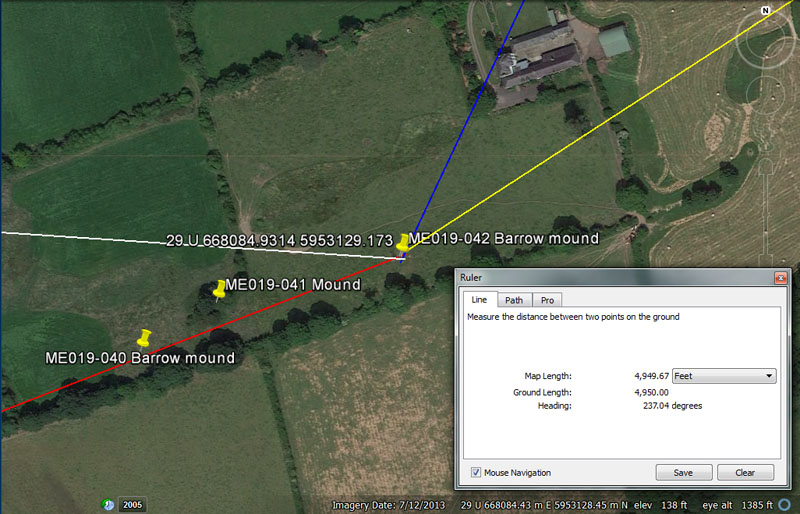Student navigators would probably have used this chambered mound for accommodation, before it became a tomb in later millenniums, as it is now thought to be.

From Knowth chambered mound (white line) the distance is 8167.5' @ 93.75-degrees.

This distance is 1/16000th of the 24750-mile (130680000') equatorial circumference or 1.35-minutes of arc (6050' under that navigational system). It is also 1 & 3/8ths of the larger mile @ 5940'. If read as 8164.8', it would be 1/16000th of the 24883.2-mile (of 5250' ... 130636800') navigational system, as well as 81 arc seconds (@ 100.8' each).

The angle value is multi-use and can be applied to navigational and compass calculations. The sum of 93.75' would be 1/56th of the 5250' mile.

From Newgrange (red line) the distance is 5062.5' @ 68.75-degrees.

The distance aspect is lunar coding and there would be 7 periods of 50.625-days (50 & 5/8ths) in a lunar year (354.375-days).

The degree angle is for mnemonic recall of the number of miles in 1-degree of equatorial arc under the 24750-mile (of 5280') navigational system, which was 68.75-miles (363000').

From Dowth chambered mound (blue line) the distance is 1650' @ 25.2-degrees.

This distance is 1/10th of a league (16500' or 3.125-miles). It is also 300 ancient fathoms, 100 rods/perches, 25 chains, 2.5 furlongs/furrowlongs or 5/16ths of a 5280' mile.

The angle accentuates one of the most important calculation values of antiquity, used in navigation. The sum of 252' would be 1/24th of 1-minute of arc, as well as 240 (what became) Greek feet of 12.6" each. A length of 25.2' would be 1/4th of 1 arc second under the 24883.2-mile (of 5250') navigational system.

From Dowth Henge (yellow line) the distance is 4950' @ 57.024-degrees return (alternative reading 56.7-degrees).

A distance of 4950-miles (of 5280') would be 1/5th of the 24750-mile equatorial circumference or 72-degrees of arc. A distance of 4950' would be .9375 (15/16ths) of a 5280' mile

The chosen angle to deliver orienteers from Dowth Henge to the mound was, undoubtedly, 56.7-degrees, encoding a strong lunar value. For example, there would be 12 periods of 567-days in the 6804-day lunar nutation cycle. or 4.5 intervals of 567-days in the 2551.5-day (7.2 lunar years) monitored within the lunisolar Sabbatical calendar. Likewise, there would be 6.25 intervals of 56.7-days in a lunar year.

It's possible that the ancient surveyors, laying out this position, would have also used the somewhat obscure coded angle of 57.024-degrees.

It's of interest to note that somewhere in the near vicinity of these 3 mounds pictured, but standing to the South near the fence-line, was a very ornately incised standing stone (archaeological reference ME019-041001). It was removed from its position after having been tumbled into the fence line ditch and now stands by the roadway directly South of Newgrange chambered mound.

If we knew its exact former position near these 3 mounds, then the codes of position or purpose of the large stone could, undoubtedly, be worked out.

This 6' 6" high X 4' wide at the base stone is incised with a single spiral of 5 revolutions, an identifiable maze pattern and two other more faded patterns that are probably mazes, a serpent pattern with a cup-mark head, another cup-mark and a well-made spiral of 8 revolutions, as well as other artistic features.

Continue